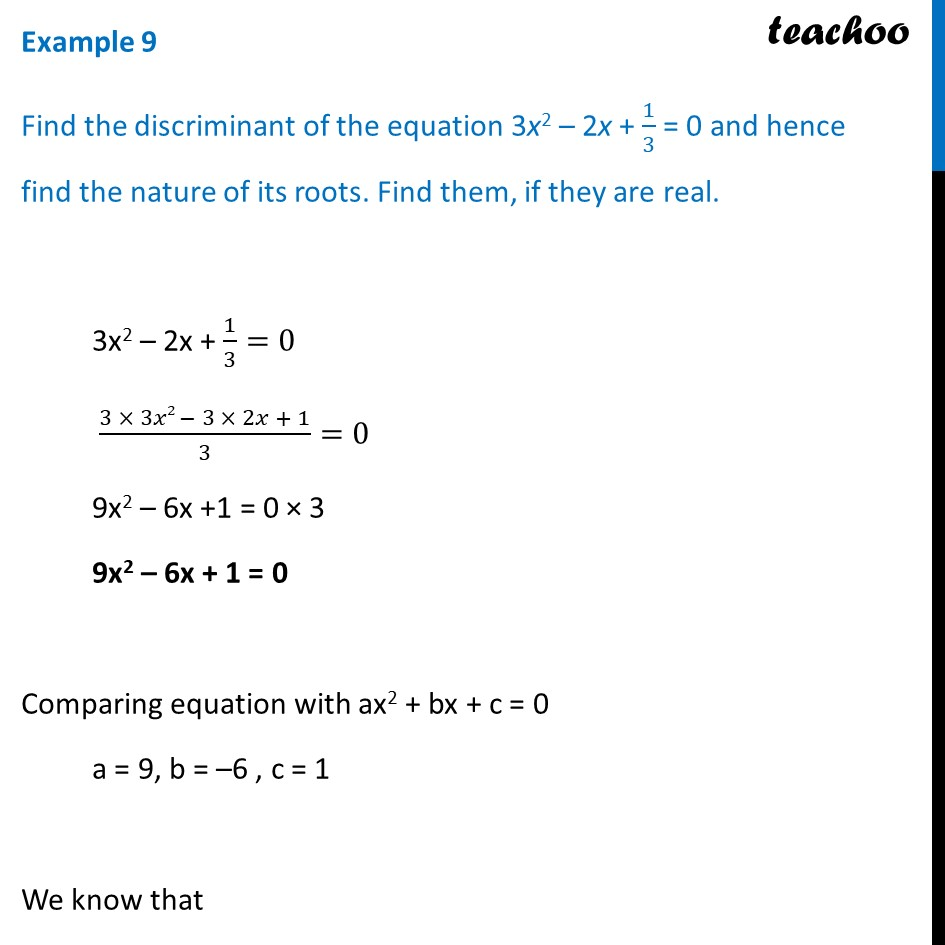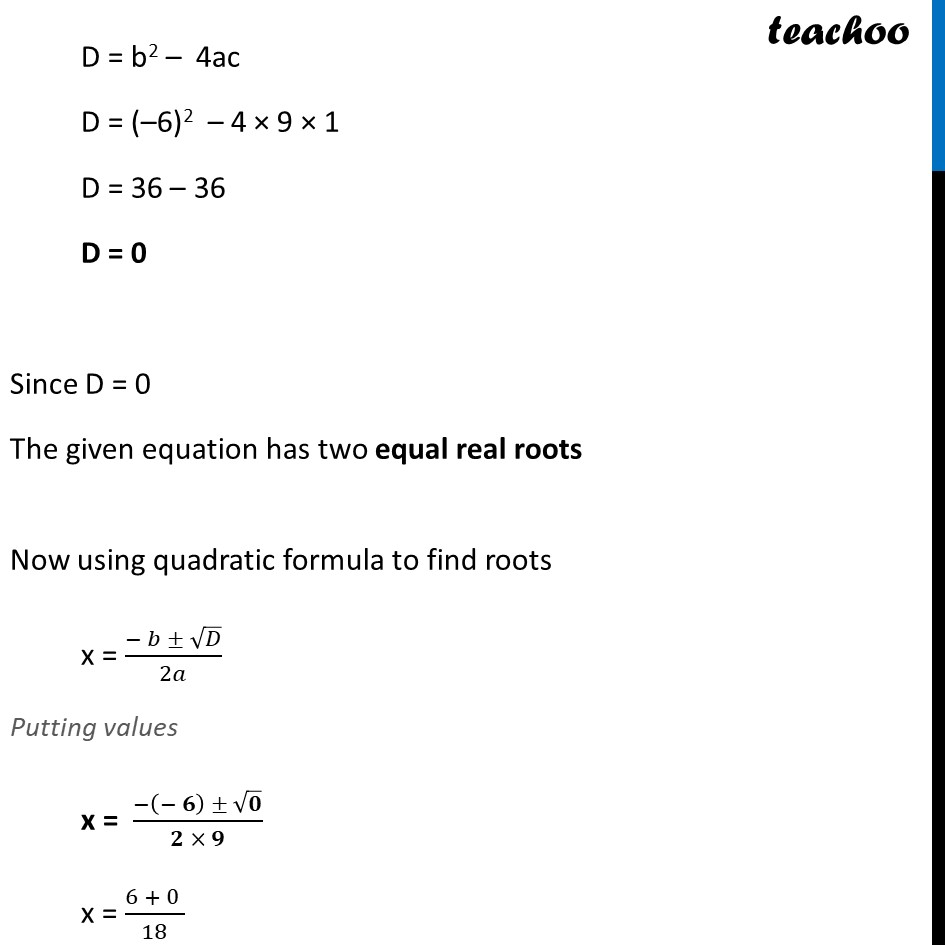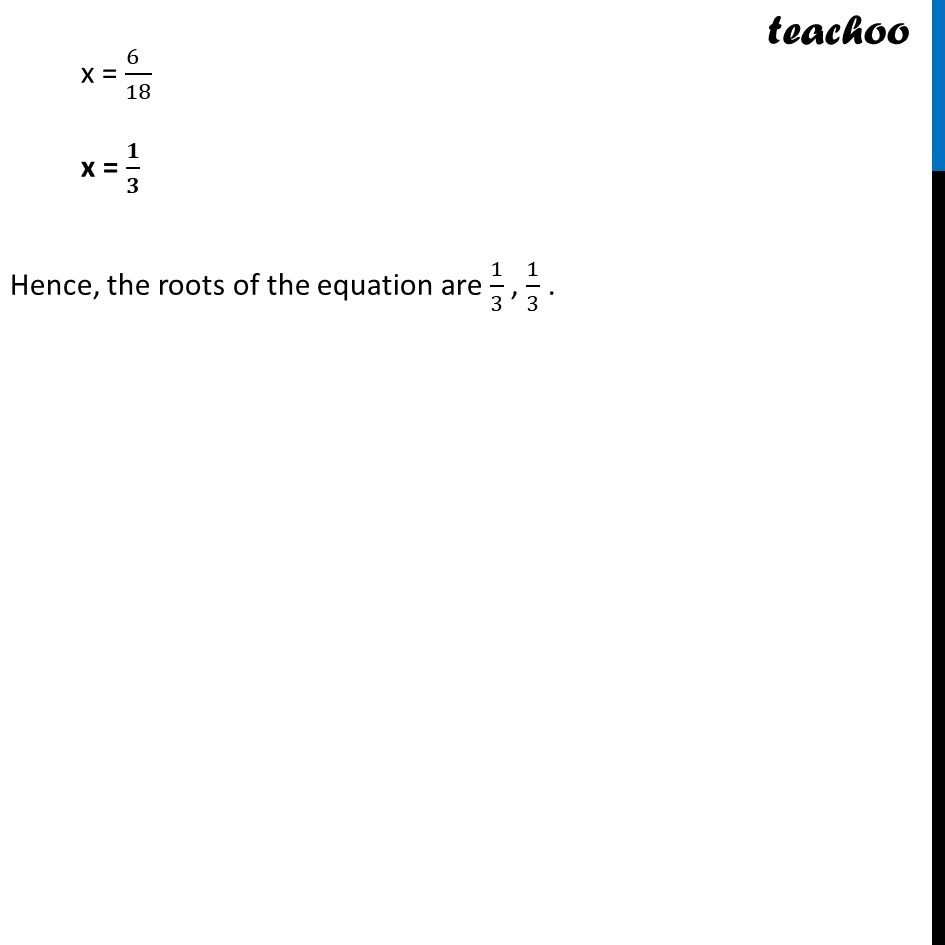Examples

Chapter 4 Class 10 Quadratic Equations
Serial order wiseLearn in your speed, with individual attention - Teachoo Maths 1-on-1 Class

### Transcript

Example 9 Find the discriminant of the equation 3x2 – 2x + 1/3 = 0 and hence find the nature of its roots. Find them, if they are real. 3x2 – 2x + 1/3=0 (3 × 3𝑥2 − 3 × 2𝑥 + 1)/3=0 9x2 – 6x +1 = 0 × 3 9x2 – 6x + 1 = 0 Comparing equation with ax2 + bx + c = 0 a = 9, b = –6 , c = 1 We know that D = b2 – 4ac D = (–6)2 – 4 × 9 × 1 D = 36 – 36 D = 0 Since D = 0 The given equation has two equal real roots Now using quadratic formula to find roots x = (− 𝑏 ± √𝐷)/2𝑎 Putting values x = (−(− 𝟔) ± √𝟎)/(𝟐 × 𝟗) x = (6 + 0 )/18 x = (6 )/18 x = 𝟏/𝟑 Hence, the roots of the equation are 1/3 , 1/3 .